There are all sorts of resonances around us, in the world, in our culture, and in our technology. A tidal resonance causes the 55 foot tides in the Bay of Fundy. Mechanical and acoustical resonances and their control are at the center of practically every musical instrument that ever existed. Even our voices and speech are based on controlling the resonances in our throat and mouth. Technology is also a heavy user of resonance. All clocks, radios, televisions, and gps navigating systems use electronic resonators at their very core. Doctors use magnetic resonance imaging or MRI to sense the resonances in atomic nuclei to map the insides of their patients. In spite of the great diversity of resonators, they all share many common properties. In this blog, we will delve into their various aspects. It is hoped that this will serve both the students and professionals who would like to understand more about resonators. I hope all will enjoy the animations.

Origins of Newton's laws of motion

History of mechanical clocks with animations
Understanding a mechanical clock with animations
includes pendulum, balance wheel, and quartz clocks

## Friday, July 12, 2013

### 3.19 - Applying methods of 3.18 to the circuit of 3.17All postings by author previous: 3.18 Separating out the radiated and simply reflected components up: Contents next: 3.20 Wave properties for transmission lines

3.19 - Applying methods of 3.18 to the circuit of 3.17

Summary: This posting completes the analysis of the two output channel circuit by applying the methods of the previous posting, i.e. 3.18 to the circuit in 3.17 .

Keywords: LRC resonator, radiated waves, transmitted waves, transmission line, absorbed power

Topics covered in this posting (basically the same as in the previous posting but applied to a different circuit)

• A review of the defining system of differential equations for a resonant LRC circuit as excited with waves on a transmission line.
• A look at the numerical solutions for the differential equations.
• Separation of the circuit into two parts, one appropriate for the radiated waves and one appropriate for the simply reflected waves.
• A look at the differential equations for both components and numerical solutions for each.
• A showing that the sum of the radiated and the simply reflected waves equals the total voltage for the initial circuit. This is done algebraically and graphically.
• A discussion of the concept of canceling waves as a method of absorbing power.1. The general setupFig. 1. LRC resonator excited by waves on a transmission line with two output channels (reflection and transmission). The physical size of the components ( i.e. V, R0 , LC , L, C and R ) is assumed to be much smaller than the length of the transmission lines and also much smaller than a wavelength of the waves traveling on the transmission lines.

This posting is similar to the last posting, except that the transmission line circuit is a little different as shown in fig. 1 at the right (compare with fig. 1 of the last posting which is missing the output transmission line and the rightmost R0  shown at the right). This posting is the final mathematical analysis of the scattering circuit shown in previous animations in postings 3.15 and 3.17.

Because this posting parallels the previous posting, it is rather minimalistic to avoid repeating a lot of the verbiage of the previous posting. The reader should examine the previous posting for a more detailed discussion of various analytic steps.

2. Equivalent circuit, differential equations, and numerical solution
Equivalent circuit for differential equation analysisFig. 2. Equivalent circuit for generating the differential equations for the transmission line circuit shown in fig. 1 above. The transmission line of characteristic impedance R0 and wave source of amplitude Vinc are replace with an AC voltage source VS = 2Vinc  and resistor R0  in series. The output transmission line (of impedance R0 ) with terminating resistor R0 are replaced by a simple resistor R0 . We are interested in calculating the incident, simply reflected and radiated waves at point A.Fig. 2 shows the equivalent circuit of the transmission line circuit of fig. 1 from the point of view of the upper end of the coupling inductor LC , point A in Fig. 2. The defining differential equations of the circuit are: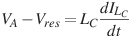(2)     voltage drop across coupling inductor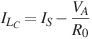(3)     conservation of current at point A(4)     voltage drop across source resistor

The above equations can be combined to yield the following differential equations: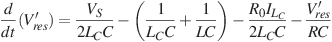(5)

Equations (5) - (7) are interactive and must be solved as a set. Equation (8) uses the solution of eqns. (5)-(7) to calculate VA ,  which is the result we are most interested in.

Below is the numerical solution of these equations in response to V  being a burst of a sinusoid at the resonant frequency. The resonant frequency was taken from 3.17 fig. 9.

Graphs based on numerical solutions to Eqns. (5) through (8)
Graph of Vres versus time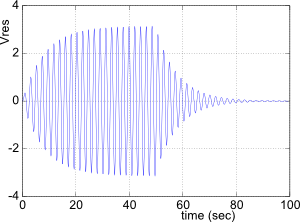Graph of VA versus timeFig. 3a. Voltage of the resonator Vres  as a function of time as solved by the lsode function in Octave. To make this graph we set LC = 5.5H and ω = 2.91rad/sec as required for unity coupling to maximize the resonator response (see posting 3.17 for details). The other circuit parameters are chosen the same as in fig. 5 of posting 3.17.

The incident waveform, not shown here, was the same as the blue trace in fig 3a of posting 3.18. The incident wave form is also shown below in fig. 5a.

Fig. 3b. Voltage at point A as a function of time as calculated with eqn. 8 above. VA also equals the total transmitted waveform, that which goes on to the right terminating resistor in Fig. 1 above.

Note that the vertical scale on this graph is different than that in fig. 3a to the left and that VA is about one quarter the amplitude of Vres .

Graph of VnegGoing versus time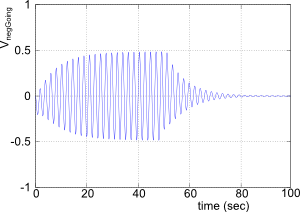The differential equations (1b), (1c) and (3) are sufficient to calculate all voltages and currents in the circuit of Fig. 1 above, including Vres  and VA  as plotted in Figs. 3a and 3b.

To calculate Vinc in Fig. 3a  we assumed that Vinc = VS/2 , as we proved in the previous posting. For the graph in Fig. 3c  we used the fact that the voltage at point A is the sum of the incident wave and the negative going wave meaning that VnegGoing = VA − Vinc . Also, the transmitted wave equals VA .

↑ Fig. 3c. Voltage of the negative going wave at point A as a function of time. This graph has the same vertical scale as fig. 3b. We see that VnegGoing has a similar amplitude as does the waveform in fig. 3b  i.e. similar to that of the total transmitted waveform traveling on the right transmission line.3. Separating out the wave components

As we did in the previous posting we separate the total voltage at point A into two components. These are:

1. The simply reflected component which equals the incident wave with the appropriate phase shift, and
2. The radiated component that originates from the resonator's oscillations.

For more details, see section 3 of the previous posting. Below are the partial circuits, modified from fig. 2 above that are appropriate for this discussion.

Circuit for modeling the incident and simply reflected waves Circuit for modeling the radiated waves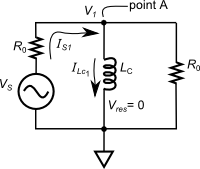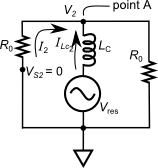↑ Fig. 4a. Circuit for modeling the simply reflected waves, with the resonator in fig. 2 shorted out and deleted. Note that we define the voltage at point A in this circuit as V1 and the current from the source as IS1 and the current through the coupling inductor LC  as  ILc1 . ↑ Fig. 4b. Circuit for modeling the radiated waves with source voltage in fig. 2 set to zero and deleted. The resonator powers the circuit similar to its action in a decay mode. Note that we define the voltage at point A as V2 , the current from the source as IS2 and the current through the coupling inductor LC  as  ILc2 . We define the direction of ILc2  in the reverse direction as in Figs. 2 and 4a.

The defining equations for the above circuit fragment (for the incident and simply reflected wave components) are:

By differentiating (11) and combining this with (10) we end up with the first order differential equation:The equation for the above circuit fragment (for the radiated wave component) is: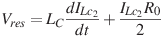.   (13)

This can be rearranged as a first order differential equation as:

Equation (14) depends on the resonator voltage Vres  and is probably best solved along with (5) through (7) above. We can use the result of (14), i.e. ILc2 , to get V2 using the equation:

Graphs of V1 and V2← Fig. 4c. V1 in blue and V2 in green as a function of time as per the equations (12), (14) and (15) just above. V1 is the voltage at point A due to the source VS (without the resonator) while V2 is the voltage at point A due to the resonator (without the source). For Vres as needed by (14) we used the solution obtained in section 2 above. We used the same circuit parameter values as used in fig. 3a above.

V1 is a slight modification of the incident signal. The oddity at the beginning and end of the burst is caused by the loading and unloading of the coupling inductor as included in fig. 4a above.

V1 also equals the simply transmitted signal which travels down the right transmission line in the absence of resonator excitation.

V2 is also the radiated signal which travels both to the left and to the right on the two transmission lines.

The sum of V1 and V2 equals the total signal traveling down the right transmission line.

The sum of V2 and Vrefl equals the total negative going wave traveling in the left transmission line. Vrefl is the wave reflected from the resonator when the resonator is not excited. It will be derived in the next section.

Fig. 4c at the left looks very similar in shape to the equivalent graph Fig. 4c of the previous posting, the biggest difference being that in the previous graph both voltages V1 and V2 are twice as large as they are in the present graph. The higher values of the previous posting are due to the abrupt end of the transmission line at the coupling inductor in the circuit of that posting and the high impedance of the coupling inductor. This causes nearly a 100% reflection at point A which effectively doubles the voltage at this point, both that due to the source and the response of the resonator. The circuit being analyzed in the current posting has a continuing transmission line to the right of point A which does not cause a noticeable reflection and hence no doubling.

The next section separates the two components of V1 . These two components are the incident and simply reflected signals.

4. Obtaining the incident and the simply reflected components from V1

The math from section 4 of the previous posting (posting 3.18) was derived based on circuit details including point A and everything to the left of point A, i.e. the part of the circuit that is the same between the circuits for the previous posting and this posting. Thus we can simply use the results of section 4 of the previous posting here. The results of that work were:

Below we graph the incident and simply reflected waveforms:

Graphs of incident and simply reflected waveforms as per eqn. (16)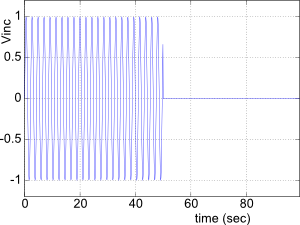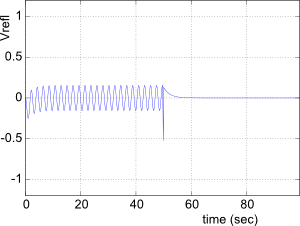Fig. 5a. The incident wave as given by (16a). This graph looks the same as it did in the previous posting and it should: it is simply VS/2 as per (16a). It shows the time dependence of the burst that eventually excites the resonator.

Fig. 5b. Simply reflected wave as given by (16b). This graph shows that the simply reflected wave is small because there is only the fairly high impedance coupling inductor hanging off the side of an otherwise continuous transmission line. Furthermore, the impedance of the coupling inductor is imaginary making its reflection 90degrees out of phase with the incident wave, so that it affects V1 in a minimal way. Thus, as we see in Fig. 4c above, V1 which is the sum of the incident and simply reflected waves at point A is almost equal to the incident wave.

Vrefl in the above graph is very different from that in the previous posting. In the previous posting Vrefl almost equals the incident wave and had a very large effect on V1, i.e. in the setup of the previous posting Vrefl doubled V1 .

5. Showing that the sum of the components V1 and V2 equal the voltage VA of the complete circuit.
Comparison of (V1 + V2) and VAFig. 6. Comparison of the sum of the component voltages (V1 + V2), in blue, and the total circuit voltage VA calculated with Eqns. (1b) through (3), in green. The sum voltage has been vertically offset to so it isn't totally covered by the other waveform.

The parameters used in making the graph were chosen the same as in Fig. 3a above. The graph was produced using Octave.

This section parallels the work in section 5 of the last posting 3.18.

We start by substituting into (11)  Is1 = ILc1  + IRo = ILc1  + V1/R0 and differentiating the result to get:

Equation (14) from above is:

We subtract (18) from (17) to get:

Since the last term in (19) equals V2/LC  (19) becomes:

Since VA = V1 + V2 and ILc = ILc1 − ILc2  (20) becomes:which is the same as equation (2) above, one of the defining equations for the whole circuit.

The other defining equations are much easier. Equation (1) defines the resonator's response and nothing to do with our breaking the circuit into pieces in section 3, since in section 3 we replaced the resonator with a unspecified voltage source but presumably governed by (1). Equations (3) and (4) are rather trivial and come from adding similar equations for the two partial circuits similar to what was done just above.

This shows conclusively that the components are consistent with the defining equations of the original circuit.

As further proof, in Fig. 6 we graphically compare numerical solutions of (V1  + V2 ) and VA . One curve has been slightly shifted so that one curve doesn't totally cover the other one. They are identical.

6. Power absorption via canceling radiation
Scattering channelsFig. 7. Abstract view of the process of a resonator being driven by waves, illustrating the concept of scattering channels. The setup in this posting would be classified as having one input channel and two output channels.

In the abstract sense, the setup in this posting (see Figs. 1 and 2) can be drawn as shown in Fig. 7 with waves coming in through one "channel" and leaving through two other "channels" (since it is straightforward to separate the positive and negative going waves of the transmission line supplying the power). The power that is absorbed internally by the resonator is the difference between that in the input channel and the output channels:

where the power in the negative going channel is given by:

and that in the transmitted channel is given by:

Without the resonator excited and if the coupling inductor has reasonably large impedance, the simply reflected wave is fairly small and will carry off minimal power. Without the resonator excited, most power will go straight through to the transmitted channel.Suppose we wish to maximize power absorbed by the resonator. According to (22), this requires minimizing the power in the waves in the two output channels. When the resonator becomes excited, most of the negative going wave is due to that radiated by the resonator. So to reduce the power in the negative going output channel we need to reduce the resonator's radiation, which means coupling more weakly to the resonator. At the same time, to reduce the power in the transmitted channel, we need reasonable coupling so that the resonator radiates sufficiently to cancel the transmitted wave.

Thus considering the backward direction, radiation should be minimized, while in the forward direction, it is essential to radiate a significant canceling wave. So to maximize power absorbed by the resonator we need to compromise and set the coupling and radiated power at a level that is not too large or too small. This is essentially what the graphs in fig. 11 of posting 3.17 tell us, that there is an optimal coupling to maximize the excitation of the resonator.

This chain of logic also tells us that there is no coupling strength that results in all the incident power being absorbed by the resonator. That is, we need some radiated wave to cancel some of the transmitted wave. On the other hand, any radiated wave will result in increased loss of power in the backward direction. Indeed, fig. 11 of posting 3.17 shows us that the best we can do is 50% absorption of power by the resonator.

The graphs in Fig. 8a and 8b below show the two wave components in the forward or transmitted output channel. Of particular note is Fig. 8b which is an expanded view of the phasing between the simply reflected and radiated wave components from Fig. 8a. We see that these two components are phased exactly 180degrees out of phase, a phasing that allows them to cancel to the maximum extent possible. Study the captions for more details of these graphs.← Vtrans (blue) and Vrad (green)

Fig. 8a. The two components that make up the total wave on the right side of the resonator. The simply transmitted wave Vtrans  (blue) is the incident wave which passes on to the right transmission line when the resonator is not excited. It closely resembles the incident sinusoidal burst.

The radiated component (green) is proportional to the resonator oscillations so we see the expected build up of oscillations followed by the decay after the burst is turned off.← Expanded view: Vtrans (blue) and Vrad (green)

Fig. 8b. An expanded view of Fig. 8a above.

The most striking feature of this graph is that the two sinusoids are perfectly out of phase. This allows the radiated component to cancel the simply reflected component to the maximum extent possible given the amplitudes of the two waves. In a sense this is one of the conditions for having the excitation right on resonance ... to minimize the negative going wave and thus maximize the power transfer to the resonator.CONCLUSION: Similar to what we saw in posting 3.18 we see above that the radiated wave is phased to cancel the simply transmitted wave to the maximum extent possible and maximize the power available to the resonator. Although we didn't show this in our graphing, the perfect cancelling phasing only occurs when the source frequency matches the resonator's frequency (with coupling inductor attached). The radiated wave is a canceling wave.

 All postings by author previous: 3.18 Separating out the radiated and simply reflected components up: Contents next: 3.20 Wave properties for transmission lines